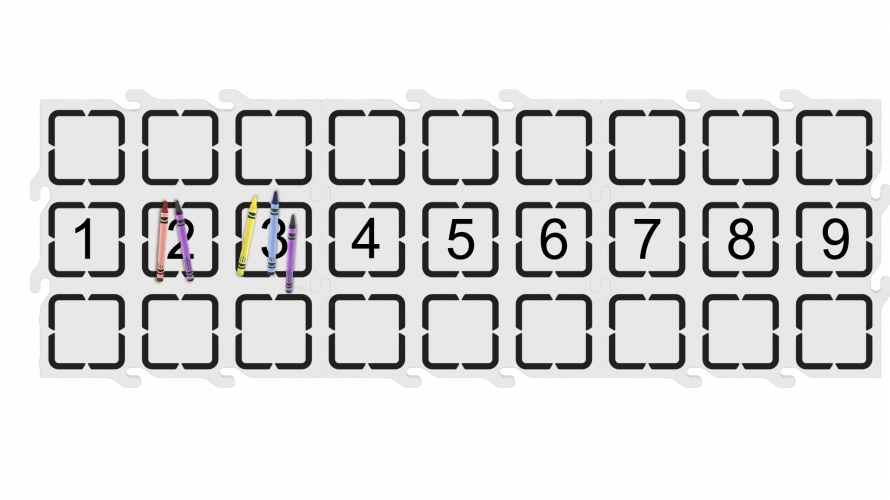Number Line
Lab 2 - Number Values

Teacher Portal

# Engage

## Launch the Engage Section

ACTS is what the teacher will do and ASKS is how the teacher will facilitate.

1. Make a personal connection to modeling and solving equations by having kids represent numbers using their fingers.
2. Model the equation (1+3=4) using your fingers. Hold up 1 finger on one hand and 3 fingers on the other hand as you ask the students the equation (1 + 3).
3. Solve an additional problem using your fingers. Count aloud each finger to solve the second equation. Emphasize the solution- the sum is 5, the number 5=5 fingers.
4. Reinforce the connection between numbers and the quantity of objects.
5. Bring students around a number line and place the correct amount of crayons next to the number line, starting at 1 and ending at 9. Point to the crayons on the number line.
1. How many is 4? Can you show me with your fingers? (Encourage students to show 4 fingers)
2. Can we add with our fingers? (Show 1 fingers, plus 3 fingers equals 4 fingers.)  How many is 1 plus 3? We just solved an equation!
3. Let’s solve another equation. Write or say 2 + 3 = ??? Let’s solve an addition problem using our fingers, two, plus three, equals what? (5)
4. How many fingers do we have in the end. Count with me, 1, 2, 3, 4, 5 (students count with you). 2+3=5. 5 = 5 fingers.
5. I’m going to represent each number on the number line with crayons next to the number that shows on the number line. Instead of using our fingers to represent numbers like we did earlier, we are going to use crayons to represent numbers.

## Engage

1. InstructInstruct students that they will be using manipulatives (crayons and fingers) to help them solve addition problems.

Start by having students solve an addition problem using their fingers as numbers. This can be the same problem or a different problem than the ones in the Acts and Asks section.

2. DistributeDistribute crayons to each group and have each group place the crayons on their number line. The number of crayons should be equal to the number on the number line.Crayons on Number Line
3. FacilitateFacilitate a class discussion about numbers and how they can be represented: fingers and crayons (or other manipulatives).
4. OfferOffer students help as they add the crayons or other manipulatives to the number line.Teacher Troubleshooting

### Facilitation Strategies

• During the environment setup, the teacher can set up each group’s number line with 123 Tiles and the dry erase markers before class. Or, the students can complete the setup for more practice in creating a number line and writing numbers.
• Tailor the addition problems given to the students in the classroom. Depending on their previous experience with math problems and number lines, students will have differing abilities and familiarity with addition.
• Students will be using their fingers in the Engage section to make a connection. Beyond the Engage section, they should not be using their fingers to count or help solve addition problems anywhere else in the Lab. Encourage students to use the crayons (manipulatives) to count and help solve addition problems.
• Use printables as manipulative to support project planning - See the printable resources available in the VEX Library, and use them with students as they are planning and building their touch projects. You could cut out the touch button symbols and have students lay them out on their desk as they build their projects, or use the color-in sheet for students to color in the sequence of button presses in their projects.